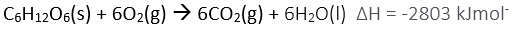Specific heat capacity exercises A brand of RAZMAN'S jelly beans was analysed for its energy content. A jelly bean of mass 1.5g was burnt completey in a bomb calorimeter containing 100ml of water. If the temperature rose from 21.56°C to 24.5°C calculate the energy content per gram of the jelly bean. Solutions 0.1 gram of methane was completely burnt in a bomb calorimeter containing 100ml of water. If the temperature increased by 11.82°C find the heat of combustion(energy released per mole)of methane. (C=12,H=1) Solution 0.1 gram of carbon was burnt in a bomb calorimeter containing 200ml of water. If the temperature of the water increased by 3.92°C calculate the energy given off, during combustion, per mole of carbon. Solution Sugar burns in oxygen according to the equation below.The equation states that for every mole of sugar burnt 2803KJ of energy are released. A 2.6 gram sample of impure sugar was burnt in a bomb calorimeter containing 100ml of water. The temperature of the water increased from 22.0°C to 24.3°C. a)Calculate the amount of energy that was released in the burning of the sugar. b) Calculate the mole and the mass of sugar present. c) Assuming no other combustible material is present calculate the percent, by mass, of sugar in the sample. (C=12,O=16,H=1) Solution